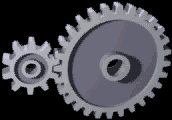# Gear

A gear (also called a cogwheel) is a type of simple machine that is used to manipulate the magnitude or direction of a force. Gears are used in combination and are linked together by their teeth - referred to as cogs - in order to form a "gear train". These gear trains are useful for transferring energy from one part of a system to another. Systems that utilize gears and gear trains include bicycles, cars, electric screwdrivers, and many other common machines.

## How they work

Gears use the principle of mechanical advantage, which is the ratio of output force to input force in a system. For gears, the mechanical advantage is given by the gear ratio, which is the ratio of the final gear's speed to the initial gear's speed in a gear train. The gear ratio is given by the equation:Figure 2: Animation of a gear pair, it can be seen that the smaller gear is spinning faster in order to keep up with the larger number of teeth on the bigger gear.

where

• is the number of teeth on the gear,
• is the angular velocity of the gear and
• is the radius of the gear.

Therefore, if the mechanical advantage of a gear train is 3, that means that the last gear in the train has a radius of 3x that of the first gear. With such a ratio, the input gear can be rotated with 3x less force than is output by the final gear, but in exchange it must be rotating 3x faster than the final gear.

This relationship for gear trains is fundamentally dependent on the law of conservation of energy. When analyzing gear trains, this concept is more easily understood by using an analysis of the conserved power of the system. This analysis relates the torques of the gears to their angular velocities. A full analysis of this type of exchange can be viewed here BROKEN LINK.

## Uses

Gears serve two main purposes: increasing speed or increasing force. In order to increase one of these, compromises must me made. For example, to increase the speed of a bicycle's wheels the force applied to the pedals must be increased. Similarly, to increase the force on the wheels the pedals must be turned faster. This technique is used when a rider is trying to climb a hill on a bike. This is all related to the conservation laws of energy and power.

Gears are of common use in many systems, but can most easily be recognized in everyday life in the cars we drive. Cars must make use of gears in order to transfer energy from the engine to the wheels efficiently and safely. An idling engine operates at about 1000 rpm - if the engine was connected directly to the wheels, this would mean the car must be moving approximately 120 kph. This means if a car's engine is turned on, it would immediately accelerate to this speed. When the engine moved into ranges with high rpm's - around 7000 - the car would be going 840 kph! Although this seems very fun, it is highly impractical. This is impractical due to the fact that a car requires a large amount of energy to get moving, so an engine trying to go full speed just as it started up wouldn't generate enough force to move the car. Therefore the car makes use of gears in a transmission, or alternatively a "gearbox", which starts off by using lower gears that generate more force in order to get the car moving, eventually moving up to higher gears that focus on speed.

The same principle of gears applies to bicycles; going uphill requires lower gears in order to supply more force to counter the force of gravity, and once the rider is back on flat land, they can switch to higher gears in order to generate more speed for their bicycle.

## For Further Reading

For further information please see the related pages below: